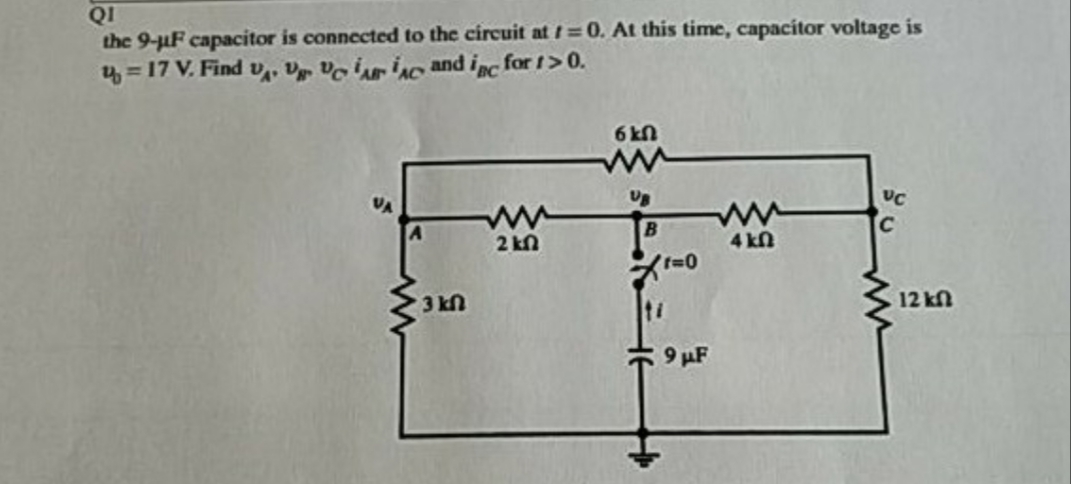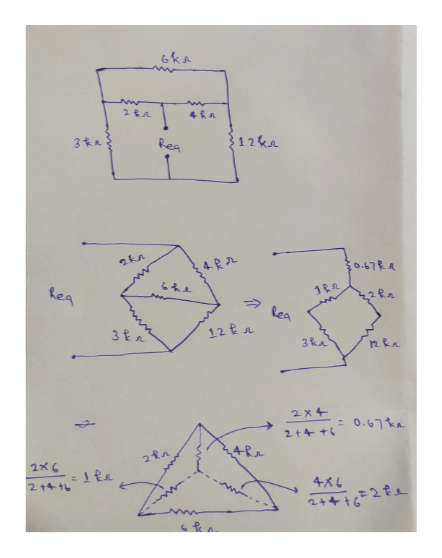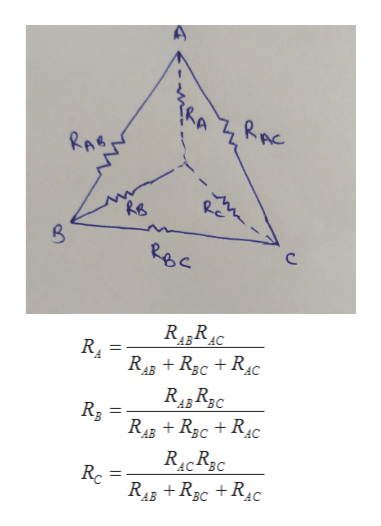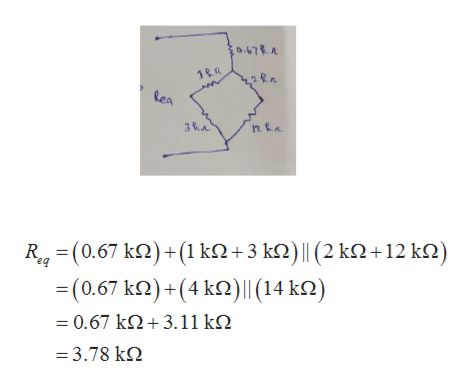# QIthe 9-uF capacitor is connected to the circuit at t = 0. At this time, capacitor voltage is4 = 17 V. Find v,, V vo iinc and inc for 1>0.6kN2 kn4 kn3 kn12 kn9 иF

Question
16 viewshelp_outlineImage TranscriptioncloseQI the 9-uF capacitor is connected to the circuit at t = 0. At this time, capacitor voltage is 4 = 17 V. Find v,, V vo iinc and inc for 1>0. 6kN 2 kn 4 kn 3 kn 12 kn 9 иF fullscreen
check_circle

Step 1

In order to calculate the time constant for the capacitor, calculate the equivalent resistance across the capacitor.

Draw a schematic diagram to calculate the equivalent resistance across the capacitor,help_outlineImage Transcriptionclose6ka {12ke Rea 3ta 0.67RA 1RR Rea a lea 12 tr 3 ta 3ka = 0.67 ka 4Re 214 16 2+416-2e fullscreen
Step 2

Wheatstone’s bridge theorem cannot be applied in figure 2 as it does not satisfy the expression of theorem.

In order to make transformation from figure 2 to figure 3, use delta-star transformation rule,

Write the expression for transformation from the delta to the star refer to the below diagram,help_outlineImage TranscriptioncloseRAC RAB. RB RBC R4„R10 R43 + R3c + R4c AB RA AB AB R3 R43 + R3c + R4c %3D AB AC Rc R43 + R3c +R4C fullscreen
Step 3

Now, apply series resistance and parallel resistance formula and...help_outlineImage TranscriptioncloseξοΑ 1tη Rea R- (0.67 ΚΩ) + (1 kD +3 kΩ) | (2 kΩ + 12 k) ba -(0.67 Κ )+ (4 kΩ) | (14 ΚΩ) -0.67 kΩ+ 3.11 kΩ =3.78 kΩ fullscreen

### Want to see the full answer?

See Solution

#### Want to see this answer and more?

Solutions are written by subject experts who are available 24/7. Questions are typically answered within 1 hour.*

See Solution
*Response times may vary by subject and question.
Tagged in

### Electrical Engineering